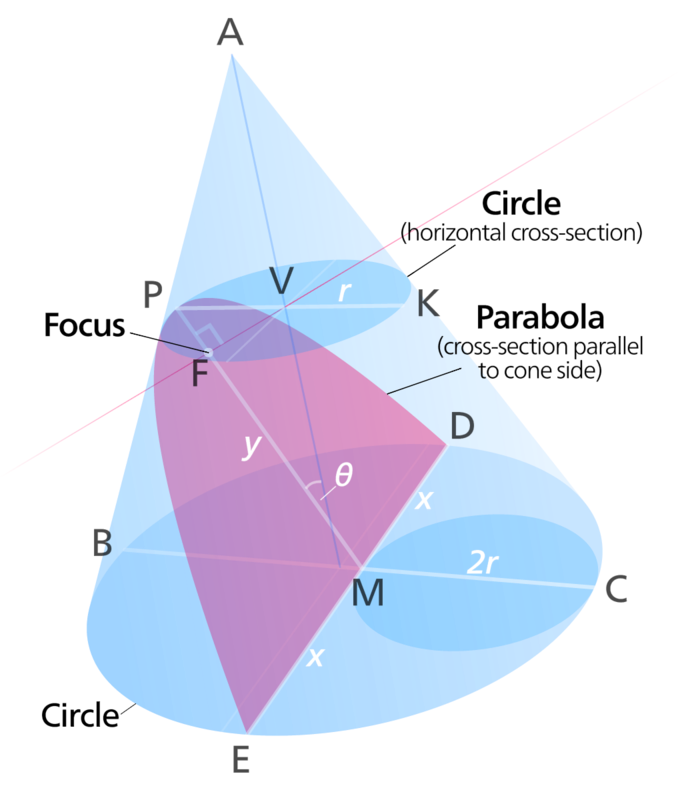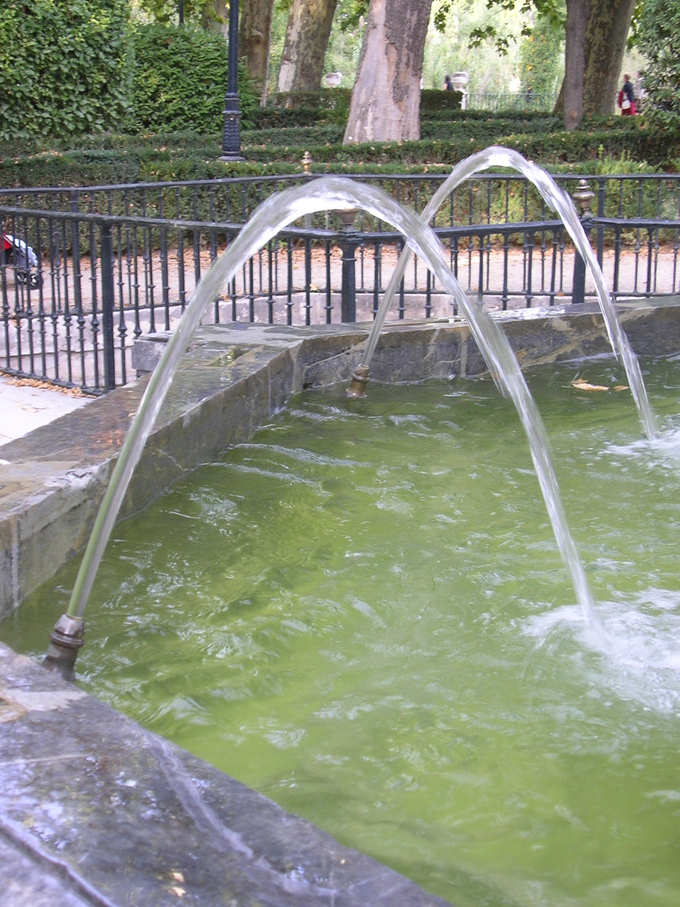## Parabolas As Conic Sections

Parabolas are one of the four shapes known as conic sections, and they have many important real world applications.

### Learning Objectives

Describe the parts of a parabola as parts of a conic section

### Key Takeaways

#### Key Points

• A parabola is formed by the intersection of a plane and a right circular cone.
• All parabolas contain a focus, a directrix, and an axis of symmetry. These vary in exact location depending on the equation used to define the parabola.
• Parabolas are frequently used in physics and engineering for things such as the design of automobile headlight reflectors and the paths of ballistic missiles.
• Parabolas are frequently encountered as graphs of quadratic functions, including the very common equation $y=x^2$.

#### Key Terms

• ballistic: Relating to projectiles moving under their own momentum, air drag, gravity, and sometimes rocket power
• directrix: A line used to define a curve or surface, especially a line from which any point on a parabola curve has a distance equal to the distance from the focus.
• focus: A point inside the parabolic section defined by forming a right triangle with the axis of symmetry and the cone’s horizontal radius.
• axis of symmetry: A line that divides the parabola into two equal halves and also passes through the vertex of the parabola.
• vertex: The point where the plane intersects the exterior surface of the right circular cone, forming one end of the parabola.

In mathematics, a parabola is a conic section, created from the intersection of a right circular conical surface and a plane parallel to a generating straight line of that surface. In other words, the plane is at the same angle as the outside surface of the cone.Parabolic conic section: This diagram shows how a parabola is generated by the intersection of a plane with a right circular cone. It also shows the $y$ and $x$ axes that would be used to view the parabola on a coordinate graph. The vertex of the parabola here is point $P$, and the diagram shows the radius $r$ between that point and the cone’s central axis, as well as the angle $\theta$ between the parabola’s axis of symmetry and the cone’s central axis.

All parabolas have the same set of basic features.
The axis of symmetry is a line that is at the same angle as the cone and divides the parabola in half.

### Vertex

The vertex is the point where the plane intersects the exterior surface of the cone. It forms the rounded end of the parabola. The vertex is therefore also a point on the cone, and the distance between that point and the cone’s central axis is the radius of a circle.

### Focus

In the diagram showing the parabolic conic section, a red line is drawn from the center of that circle to the axis of symmetry, so that a right angle is formed. The point on the axis of symmetry where the right angle is located is called the focus. By doing this, a right triangle is created.Right triangle: A right triangle is formed from the focal point of the parabola.

The focal length is the leg of the right triangle that exists along the axis of symmetry, and the focal point is the vertex of the right triangle. Using the definition of sine as opposite over hypotenuse, we can find a formula for the focal length “$f$” in terms of the radius and the angle:

\displaystyle{ \begin{align} \sin(\theta) &= \frac{f}{r} \\ f &= r\sin(\theta) \end{align} }

### Directrix

All parabolas have a directrix. The directrix is a straight line on the opposite side of the parabolic curve from the focus. The parabolic curve itself is the set of all points that are equidistant (equal distances) from both the directrix line and the focus.

### Features of Parabolas

On a coordinate plane, parabolas are frequently encountered as graphs of quadratic functions, such as:

$y=x^2$

Parabolas can open up, down, left, right, or in some other arbitrary direction. Any parabola can be repositioned and rescaled to fit exactly on any other parabola—that is, all parabolas are similar.

To locate the $x$-coordinate of the vertex, cast the equation for $y$ in terms of $a x^2 + b x + c$. The vertex will be at the point:

$\displaystyle{x= \frac{-b}{2a}}$

For example, in the parabola $y=x^2$, $a=1$, $b=0$, $c=0$, and the vertex is at $x=0$.

Parabolas have the property that, if they are made of material that reflects light, then light which enters a parabola traveling parallel to its axis of symmetry is reflected to its focus. This happens regardless of where on the parabola the reflection occurs. Conversely, light that originates from a point source at the focus is reflected, or collimated, into a parallel beam. The light leaves the parabola parallel to the axis of symmetry. The same effect occurs with sound and other forms of energy. This reflective property is the basis of many practical uses of parabolas.

The parabola has many important applications, from the design of automobile headlight reflectors to calculating the paths of ballistic missiles. They are frequently used in physics, engineering, and other sciences.

## Applications of the Parabola

Parabolas have important applications in physics, engineering, and nature.

### Learning Objectives

Discuss models of physical situations that use parabolas

### Key Takeaways

#### Key Points

• Projectiles and missiles follow approximately parabolic paths. They are approximate because real-world imperfections affect the movements of objects.
• Parabolic reflectors are common in microwave and satellite dish receiving and transmitting antennas.
• Paraboloids are also observed in the surface of a liquid confined to a container and rotated around the central axis.

#### Key Terms

• projectile: Any object propelled through space by the application of a force.
• ballistics: The science of objects that predominately fly under the effects of gravity, such as bullets, missiles, or rockets.
• conical: Shaped like a cone; of or relating to a cone or cones.

A parabola is a conic section created from the intersection of a right circular conical surface and a plane parallel to a generating straight line of that surface. The parabola has many important applications, from the design of automobile headlight reflectors to calculating the paths of ballistic missiles. They are frequently used in areas such as engineering and physics, and often appear in nature.

The parabolic trajectory of projectiles was discovered experimentally in the 17th century by Galileo, who performed experiments with balls rolling on inclined planes. What Galileo discovered and tested was that when gravity is the only force acting on an object, the distance it falls is directly proportional to the time squared. This is the exact mathematical relationship we know as a parabola.

For objects extended in space, such as a diver jumping from a diving board, the object follows a complex motion as it rotates, while its center of mass forms a parabola.

### Real World Parabolic Trajectories

As in all cases in the physical world, using the equation of a parabola to model a projectile’s trajectory is an approximation. The presence of air resistance, for instance, distorts the parabolic shape. At higher speeds, such as in ballistics, the shape can be highly distorted. This is because air resistance is a force acting on the object, and is proportional to the object’s area, density, and speed squared. For dense objects, and/or at low speeds, the air resistance force is small. So, at low speeds the parabola shape can be a very good approximation.Parabolic water trajectory: In this image, the water shot from a fountain follows a parabolic trajectory as gravity pulls it back down.

### Examples

Paraboloids arise in many physical situations. All of the physical examples are situations where an object’s trajectory or the shape of an object fits a generalized parabola function:

$y = ax^2 + bx + c$

The parameters $a$, $b$, and $c$ determine the direction as well as the exact shape and position of the parabola.

### Parabolic Reflectors

One well-known example is the parabolic reflector—a mirror or similar reflective device that concentrates light or other forms of electromagnetic radiation to a common focal point. Conversely, a parabolic reflector can collimate light from a point source at the focus into a parallel beam. This principle was applied to telescopes in the 17th century. Today, paraboloid reflectors are common throughout much of the world in microwave and satellite dish receiving and transmitting antennas.

### Liquid Paraboloids

Paraboloids are also observed in the surface of a liquid confined to a container that is rotated around a central axis. In this case, liquid moves away from the center, and it “climbs” the walls of the container, forming a parabolic surface. This is the principle behind the liquid mirror telescope.

### The “Vomit Comet”

Aircraft used to create a weightless state for purposes of experimentation, such as NASA’s “Vomit Comet,” follow a vertically parabolic trajectory for brief periods. This allows them to trace the course of an object in free fall. This can produce the same effect as zero gravity, and lets the passengers on the aircraft experience the feeling of being in space.